# Disciplined convex programming error! So, how to solve this problem in CVX?

What I want to achieve here is that S must be a positive semidefinite matrix. Just like this: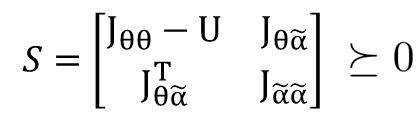Where U is an optimize variable, and it’s positive semidefinite, as: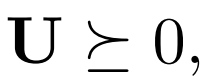J_θθ, J_(θα ̃),J_(α ̃α ̃) are the expression of another optimize variable T.

The results of some MATLAB code runs are as follows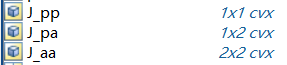Here, J_pp∈1×1 means J_θθ, J_pa∈1×2 means J_(θα ̃), and J_aa∈2×2 means J_(α ̃α ̃) .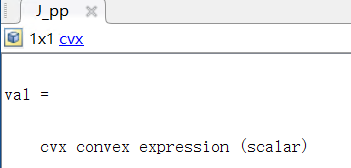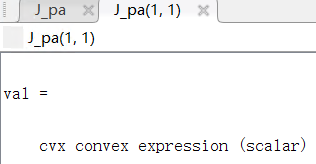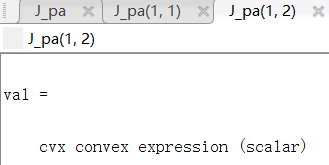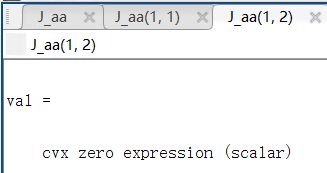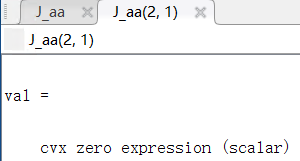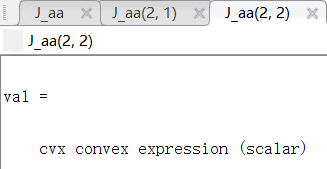And U is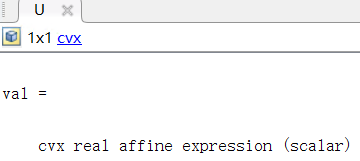My variable declaration is as follows: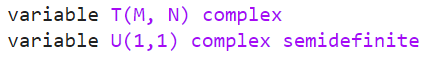When performing the program on line 41 to make S must be a positive semidefinite matrix, the following error prompt will be generated: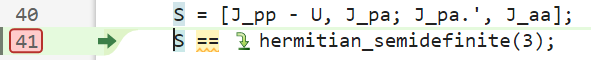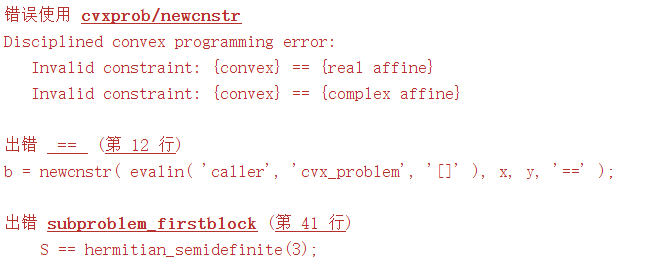Note: the function square_pos( norm(A*T,‘fro’) ) is used to generate the J_pp, J_pa and J_aa. Thus, J_pp, J_pa and J_aa are all cvx convex expression. Where A is given matrix.

And I know the reason for this error is that both sides of the equal sign must be affine.
So, Is there any way to solve this problem?

CVX requires all SDP constraints to be “linear”, i.e. affine in the optimization (CVX) variables. Yours are not. You have some kind of nonlinear SDP.

Have you proven your problem is convex? I will presume it is not unless you show otherwise.